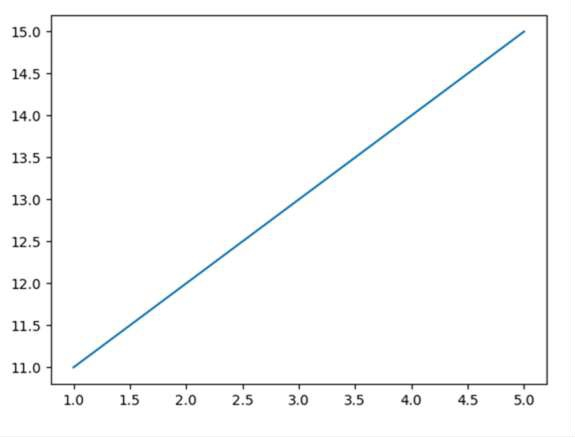# How to prevent numbers being changed to exponential form in Python Matplotlib?

Using style='plain' in the ticklabel_format() method, we can restrict the value being changed into exponential form.

## Steps

• Pass two lists to draw a line using plot() method.

• Use ticklabel_format() method with style='plain'. If a parameter is not set, the corresponding property of the formatter is left unchanged. Style='plain' turns off scientific notation.

• To show the figure, use plt.show() method.

## Example

from matplotlib import pyplot as plt
plt.plot([1, 2, 3, 4, 5], [11, 12, 13, 14, 15])
plt.ticklabel_format(style='plain')    # to prevent scientific notation.
plt.show()

## Output